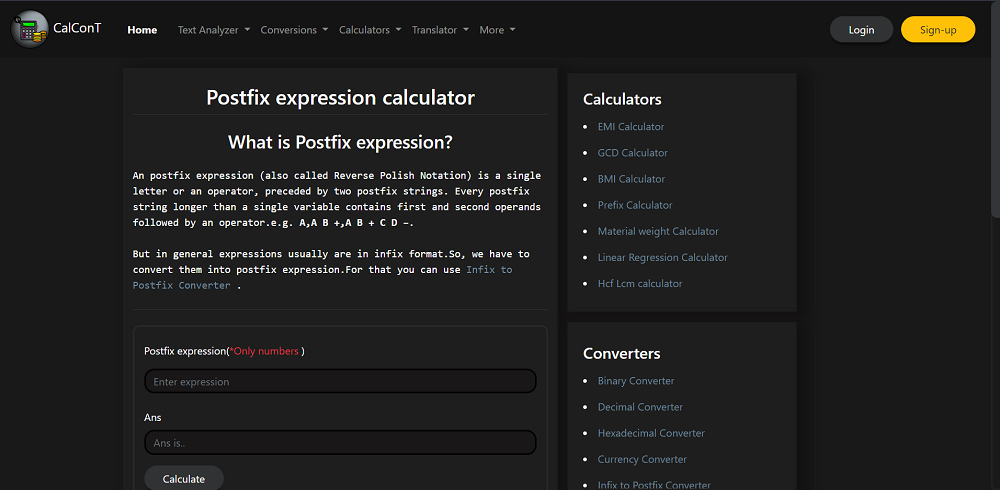# How to Build a Postfix Calculator: A Step-by-Step Guide

If you're a web developer, you've likely encountered the need for a calculator on a website. Whether you're building an e-commerce platform, a budgeting tool, or a scientific calculator, the ability to perform mathematical operations is essential. In this blog post, we'll show you how to build a postfix calculator with JavaScript so that you can easily integrate this in your web project.

###Photo by iSawRed on UnsplashWhat is a Postfix Calculator?

A postfix calculator, also known as a reverse Polish notation (RPN) calculator, is a type of calculator that uses a postfix expression to perform mathematical operations. In a postfix expression, the operator follows the operands. For example, in the expression "2 3 +", the operator "+" follows the operands "2" and "3". This expression is equivalent to the infix expression "2 + 3".

### Building the Calculator

To build a postfix calculator, we'll use HTML, CSS, and JavaScript. Here's the HTML code for our calculator form:

``````<form>
<div class="form-group my-4">
<label>Postfix expression</label>
<input class="form-control" id="Postfix" required="" type="text" />
</div>
<div class="form-group">
<input class="form-control" disabled="" id="Ans" type="number" />
</div>
<button class="btn btn-outline-secondary mb-3 text-light cal" type="submit">
Calculate
</button>
</form>
``````

This form includes an input field for the postfix expression, an input field for the answer, and a submit button. We've used Bootstrap classes to style the form.

Next, it's time to write the JavaScript code to evaluate the prefix expression and calculate the result. You can write this JavaScript in script tag within same html file or can create different js file & link that js in html file.

Here is the full code:

``````document.querySelector('.convert').addEventListener('click', function(e) {  var postfix = document.getElementById("Postfix").value;
var stack = [],
top = -1;

var i, a, b, result, pEval, ch;

for (i = 0; i <= postfix.length; i++) {
ch = postfix[i];

if (!isNaN(parseInt(ch))) {
stack[++top] = parseInt(ch);
} else if (ch == "+" || ch == "-" || ch == "*" || ch == "/") {
b = stack[top];
top--;
a = stack[top];
top--;

if (ch == "+") {
result = a + b;
} else if (ch == "-") {
result = a - b;
} else if (ch == "*") {
result = a * b;
} else if (ch == "/") {
result = a / b;
}
stack[++top] = result;
}
}

pEval = stack[top];
document.getElementById("Ans").value = pEval;
e.preventDefault();
});``````

And here it's step by step explanation with code snippets:

1. We begin by adding a click event listener to the "Calculate" button. This will execute the code when the user clicks the button:
2. ``````  document.querySelector('.convert').addEventListener('click', function(e) {
// code goes here
});
``````
3. Next, we get the user's input from the "Postfix" input field & initialize stack array, top pointer & other variables :
4. ``````var postfix = document.getElementById("Postfix").value;
var stack = [],top = -1;
var i, a, b, result, pEval, ch;
``````
5. Following line of code starts a for loop that iterates through each character in the "postfix" string.
6. ``````for (i = 0; i <= postfix.length; i++) {
ch = postfix[i];
}
``````
7. Following piece of code checks if the current character is a number. If it is, the character is converted to an integer and pushed onto the "stack" array.
8. ``````if (!isNaN(parseInt(ch))) {
stack[++top] = parseInt(ch);
}
``````
9. This section of code checks if the current character is an operator (+, -, *, or /). If it is, the two most recently added numbers are popped off the "stack" array, the operation is performed on them, and the result is pushed back onto the "stack" array.
10. `````` else if (ch == "+" || ch == "-" || ch == "*" || ch == "/") {
b = stack[top];
top--;
a = stack[top];
top--;

if (ch == "+") {
result = a + b;
} else if (ch == "-") {
result = a - b;
} else if (ch == "*") {
result = a * b;
} else if (ch == "/") {
result = a / b;
}
stack[++top] = result;
}
``````
11. Assigning evaluated postfix expression value to other input field & e.preventDefault() will helps to prevent the form from submitting to avoid page reload.
12. ``````pEval = stack[top];
document.getElementById("Ans").value = pEval;
e.preventDefault();``````
Here's how the calculator looks after some CSS modifications and using built-in Bootstrap classes. You can see a screenshot of the updated design below and test it using this link.CalConT

You can checkout how I developed this calculator in calcont's github repo & contribute to it as well.

Hope you got the idea for developing Postfix calculator using html & javascript, Thanx !!